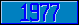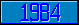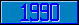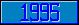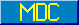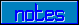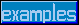## \$%CSIN

### M[UMPS] by Example

```
2-Jul–96, 6:54:35

Routine Save for Complex Mathematics Library function \$%CSIN^MATH

;

; Unless otherwise noted, the code below

; was approved in document X11/95–14

;

; If corrections have been applied,

; first the original line appears,

; with three semicolons at the beginning of the line.

;

; Then the source of the correction is acknowledged,

; then the corrected line appears, followed by a

; line containing three semicolons.

;

CSIN(Z,PREC) ;

New IA,E1,E2

;

;;;

; Alan Frank (October 1995)

Set PREC=\$Get(PREC,11)

;;;

;

Set IA=\$%CMUL^MATH(Z,"0%1")

Set E1=\$%CEXP^MATH(IA,PREC)

Set IA=-IA_"%"_(-\$Piece(IA,"%",2))

Set E2=\$%CEXP^MATH(IA,PREC)

Set IA=\$%CSUB^MATH(E1,E2)

Set IA=\$%CMUL^MATH(IA,"0.5%0")

Quit \$%CMUL^MATH("0%–1",IA)

;===

;

;

;

```